# Decision Trees — An Intuitive Introduction

An extensive introduction including a look at decision tree classification, data distribution, decision tree regression, decision tree learning, information gain, and more.

#### Decision Tree Learning

While building a decision tree it is very important to ask the right questions at the correct stage in a tree. That is what essentially building a decision tree or decision tree learning means.There are a lot of algorithms which are employed to build a decision tree, ID3, C4.5, C5.0, CART to name a few but at their core all of them tell us what questions to ask and when.

Lets run through an example to understand how a decision tree learns. The below table has color and diameter of a fruit and the label tells the name of the fruit. How do we build a decision tree to classify the fruits?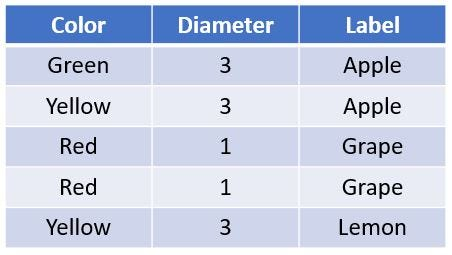Here is how we will build the tree. We will start with a node which will ask a true or false question to split the data into two. The two resulting nodes will each ask a true or false question again to split the data further and so on.

There are 2 main things to consider with the above approach -

• Which is the best question to ask at each node
• When do we stop splitting the data further

Lets start building the tree with the first or the top most node. There is a list of possible questions which can be asked. The first node can ask the following questions —

Is the color green?
Is the color yellow?
Is the color red?
Is the diameter ≥ 3?
Is the diameter ≥ 1?

Of these possible set of questions, which one is the best to ask so that our data is split into two sets after the first node? Remember we are trying to split or classify our data into separate classes. Our question should be such that our data is partitioned into as unmixed or pure classes as possible. An impure set or class here refers to one which has many different types of objects for example if we ask the question for the above data, “Is the color green?” our data will be split into two sets one of which will be pure the other will have a mixed set of labels. If we assign a label to a mixed set we have higher chances of being incorrect. But how do we measure this impurity?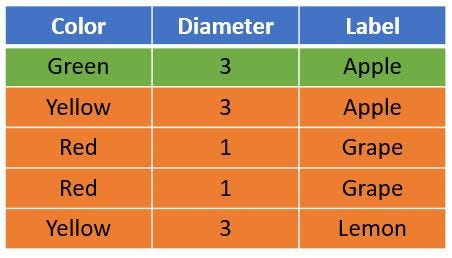Post split one set has just an apple whereas the other has Apple, Grape and Lemon

#### Gini Impurity

Gini impurity is a metric of measuring the mix of a set.The value of Gini Impurity lies between 0 and 1 and it quantifies the uncertainty at a node in a tree. Lets see what that means. Imagine two bowls — one filled with apples and the other filled with labels. If you randomly pick an object from the left bowl and assign a label from the right bowl you have no chances of being incorrect therefore the impurity is 0.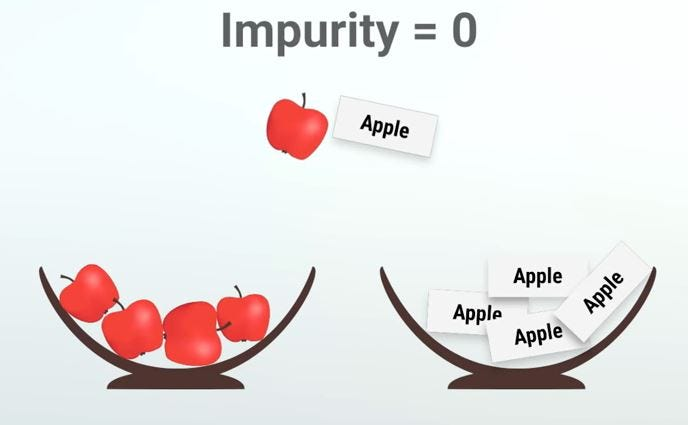On the other hand if you have a bowl with a mixture of objects and you pick an object randomly and assign a label to it you have a 1 out of 5 chance of being correct and hence 4 out of 5 chances of being incorrect which is also the Gini Impurity value.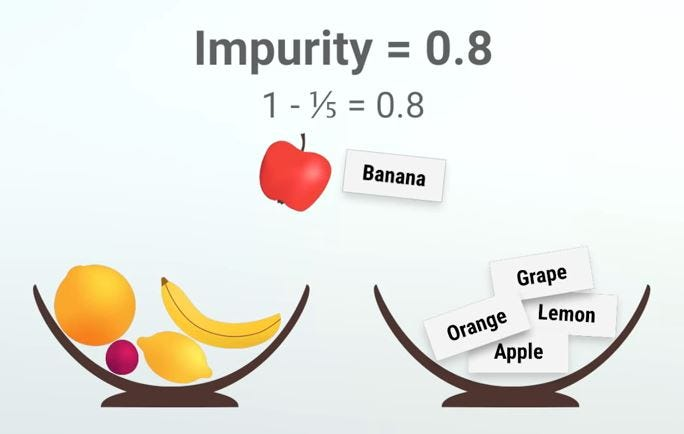So Gini Impurity tells us how mixed up or impure a set is. Now our goal with classification is to split or partition our data into as pure or unmixed sets as possible. If we reach at a 0 Gini Impurity value we stop dividing the tree further. So which question should we ask so that our data splits into as pure sets as possible?

#### Information Gain

Information gain is another metric which tells us how much a question unmixes the labels at a node. Mathematically it is just a difference between impurity values before splitting the data at a node and the weighted average of the impurity after the split. For instance, if we go back to our data of apples, lemons and grapes and ask the question “Is the color Green?”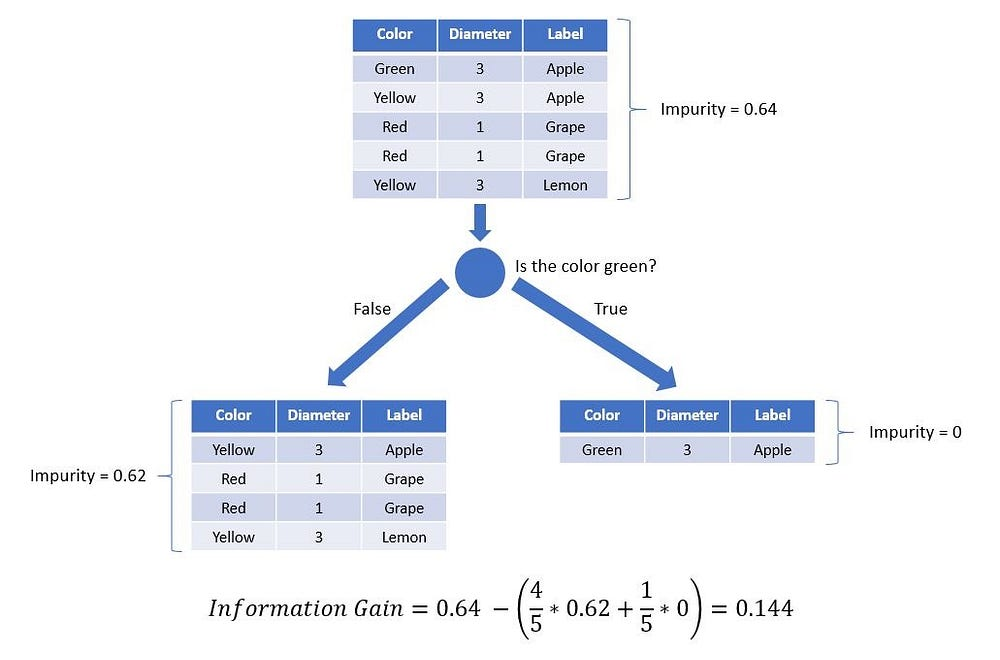Illustration of Information Gain

The information gain by asking this question is 0.144. Similarly we can ask another question from the set of possible questions split the data and compute information gain.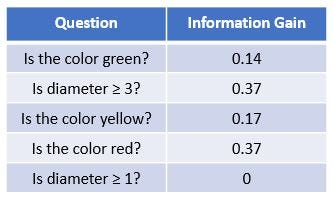The question where we have the highest information gain “Is diameter ≥ 3?” is the best question to ask. Note that the information gain is same for the question “Is the color red?” we just picked the first one at random.

Repeating the same method at the child node we can complete the tree. Note that no further questions can be asked which would increase the information gain.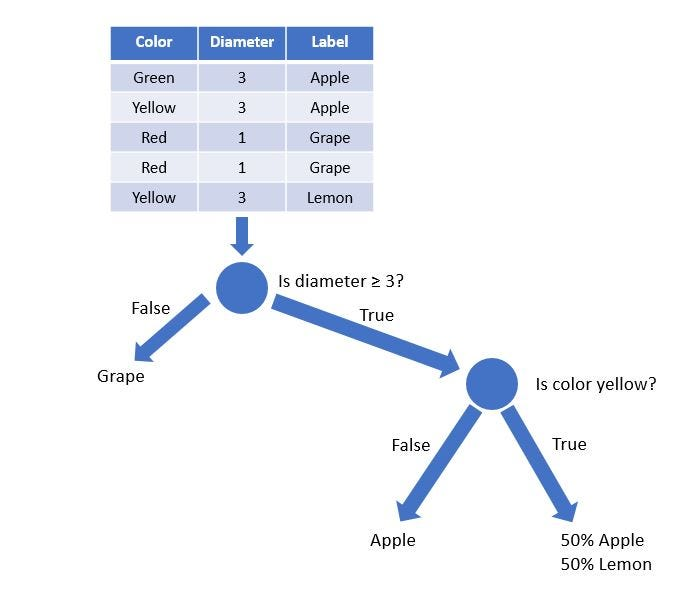Also note that the rightmost leaf which says 50% Apple & 50% lemon means that this class cannot be divided further and this branch can tell an apple or a lemon with 50% probability. For the grape and apple branches we stop asking further questions since the Gini Impurity is 0 for those.

This algorithm we used above to build the decision tree is called CART (Classification And Regression Trees). There are other algorithms which use different techniques to build a tree but the idea remains the same. What are the best questions to ask and when.

#### Final Remarks

The above introduction hopefully has elicited some curiosity though it just scratches the surface of decision trees. I would encourage you to go and explore further. Read about them and maybe code a decision tree yourself. There are a lot of resources on the internet to help you with that.

There are lot of techniques and other algorithms used to tune decision trees and to avoid overfitting, like pruning. Bagging and boosting are few techniques which when combined with decision trees make them very powerful classification tools. Although, decision trees are usually unstable which means a small change in the data can lead to huge changes in the optimal tree structure yet their simplicity makes them a strong candidate for a wide range of applications. Before neural networks became popular, decision trees were the state of the art algorithm in Machine Learning. Several other ensemble models like Random Forests are much more powerful than vanilla decision tree. We will talk about them in future articles.

Decision trees are powerful because of their simplicity and interpretability. Decision trees and random forests are highly used in user signup modelling, credit scoring, failure prediction, medical diagnostics etc. in the industry and as I said earlier we use it knowingly or unknowingly in our day to day lives as well.BioPrateek Karkare is an Electrical engineer in training with a keen interest in Physics, Computer Sciences and Biology.

Original. Reposted with permission.

Resources:

Related: